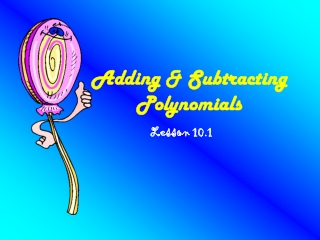DownloadDownload PresentationTélécharger la présentation- - - - - - - - - - - - - - - - - - - - - - - - - - - E N D - - - - - - - - - - - - - - - - - - - - - - - - - - -
##### Presentation Transcript

1. Adding & Subtracting Polynomials Lesson 10.1

2. Polynomial: Poly: Many nomial: terms Form: axk Where k is a non-negative integer. This is a polynomial in one variable. k is the degree ofax. ax alone has a degree of 1 The constant “a” has a degree of0.

3. Degree:the degree of a polynomial is the largest degree of its terms. Standard form:terms are written in descending order fromthe largest to the smallest degree. Coefficient:the integer in front of the variable. How many you have of each variable. If no number, you have one.

4. Put this in standard form. -4x2 + 3x3 + 2 3x3 – 4x2 + 2 Name the coefficients and degree. 2x3 + (-1)x2 + 5 Coefficients: 2, -1 Degree: 3 Coefficients: -5, 10 Degree: 2 -5x2 + 10x - 3

5. Classifying Polynomials

6. Adding Polynomials: add liketerms! You add the coefficients, not the variables! Horizontal format: (2x2+x-5) + (x2+x+6) remove ( ) = 2x2+x2+x+x-5+6 =3x2+2x+1

7. Vertical format: line up like terms and add. (2x2+x-5) + (x2+x+6) remove ( ) and line up like terms. 2x2+x-5 + x2+x+6 3x2+2x+1

8. Subtracting polynomials: use either vertical or horizontal format. ***Remember to change the signs of every term in the second polynomial when you remove the ( )! Vertical format: (8x4-3x2-11x-3) – (-13x4-3x2+2x-17) 8x4 - 3x2- 11x - 3 13x4+3x2-2x+17(combine after changing signs) 21x4 -13x+14

9. Horizontal format: (8x4-3x2-11x-3) – (-13x4-3x2+2x-17) Remove ( ) changing the signs in the second polynomial. You are adding the opposites! 8x4-3x2-11x-3 + 13x4+3x2-2x+17 (now combine like terms) 21x4-13x+14 Classify this by degree and terms. Quartic, trinomial